# C# Difference between Ref and Out Keywords with Examples

By : Suresh Dasari
Posted On : 08-May-2023

Here we will learn what is ref keyword in c#, uses of ref keyword, what is out keyword in c#, uses of out keyword, and the differences between ref and out keywords in c# with examples.

## What is Ref Keyword in C#?

In c#, while calling any function with parameters, we will send the copy of the variable to the function instead of its original value, which means the changes we do inside the function will not affect the outside variable value. To understand this, we will create a simple method example in c#.

using System;

namespace Tutlane
{
class Program
{
static void Main(string[] args)
{
int x = 10;
int y = 10;
Console.WriteLine(\$"Before Change: x = {x}, y = {y}");
ModifyValues(x, y);
Console.WriteLine(\$"After Change: x = {x}, y = {y}");
}
public static void ModifyValues(int x, int y)
{
x += 10;
y += 5;
Console.WriteLine(\$"Modified Values: x = {x}, y = {y}");
}
}
}

In this function example, we are updating the value of variables inside the method. The updates that we do inside the function will not affect the actual value of variables. When we execute the above example, we will get the result as shown below.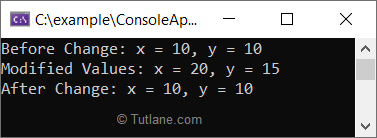If you observe the result, the changes that we did inside the function didn’t affect the outside of the variable values as we only send a copy of the variables.

If you want to change the value of variables when there is a change in our methods that can be achieved by using `ref` and `out` keywords.

In c#, the ref keyword is useful to send the reference of a variable to the method and the changes that we do inside the method will also affect the outside variable.

Basically, the functions/methods in c# will return only one value that is either an integer, string, list, etc. If you want to return more than one value, the `ref` keyword will be useful for us.

Following is the syntax of defining the variables and passing parameters to the method using the `ref` keyword in c#.

int x = 10;
int y = 10;
ModifyValues(ref x, ref y);
...
...
// Method with ref parameters
public void ModifyValues(ref int x, ref int y)
{
// implementation
}

To pass parameters using `ref` keyword, you need to explicitly define the parameters with the `ref` keyword in both the method definition and while calling the method.

Before sending parameters using the `ref` keyword, you need to remember that the variables that are passing as method arguments using the `ref` keyword must be initialized before sending it to the method otherwise, we will get the “Use of unassigned local variable” compilation error as shown below.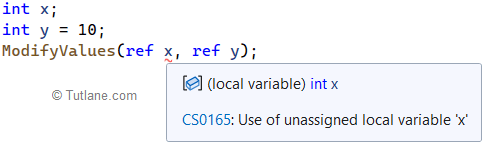## C# Ref Keyword Example

Following is an example of using the `ref` keyword to send parameters to the method as reference type and return the multiple values from the method in c#.

using System;

namespace Tutlane
{
class Program
{
static void Main(string[] args)
{
int x = 10;
int y = 10;
Console.WriteLine(\$"Values Before Calling Method: x = {x}, y = {y}");
ModifyValues(ref x, ref y);
Console.WriteLine(\$"Values After Calling Method: x = {x}, y = {y}");
}
public static void ModifyValues(ref int x, ref int y)
{
x += 10;
y += 5;
Console.WriteLine(\$"Modified Values Inside Method: x = {x}, y = {y}");
}
}
}

In this ref parameter example, we explicitly specified the variables using the ref keyword in both the method definition (ModifyValues) and while calling the method.

When you execute this example, you will get the result as shown below.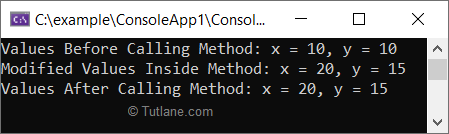If you observe the result, the changes that we did inside the method have affected the variables that are outside of the function, and we are able to return the multiple values from the ModifyValues method.

## What is Out Keyword in C#?

In c#, the `out` keyword is same as the `ref` keyword to pass method arguments as reference types, but the only difference is you don’t need to initialize the variable that you want to pass as a method argument but the method must assign a value to the variable before it returns the value.

Following is the syntax of defining the variables and passing parameters to the methods using `out` parameter in c#.

int x;
int y = 10;
ModifyValues(out x, out y);
...
...
// Method with out parameters
public void ModifyValues(out int x, out int y)
{
// implementation
}

Same as `ref` keyword, to pass parameters using `out` keyword you need to explicitly define the parameters with `out` keyword in both the method definition and while calling the method.

Before passing parameters using the `out` keyword, you need to remember that the variables that are passing as method arguments using the `out` keyword must be initialized before sending it to the method otherwise, we will get the “Use of unassigned local variable” compilation error as shown below.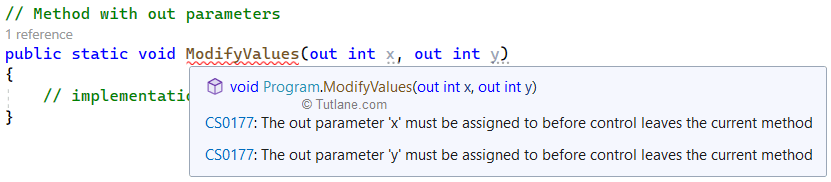## C# Out Parameter Example

Following is an example of using `out` parameter to send parameters to the method as reference type and return the multiple values from the method in c#.

using System;

namespace Tutlane
{
class Program
{
static void Main(string[] args)
{
int x = 10;
int y = 10;
Console.WriteLine(\$"Values Before Calling Method: x = {x}, y = {y}");
ModifyValues(out x, out y);
Console.WriteLine(\$"Values After Calling Method: x = {x}, y = {y}");
}
public static void ModifyValues(out int x, out int y)
{
x = 20;
y = 25;
Console.WriteLine(\$"Modified Values Inside Method: x = {x}, y = {y}");
}
}
}

In this `out` parameter example, we explicitly specified the variables using the `out` keyword in both the method definition (ModifyValues) and while calling the method.

When you execute this example, you will get the result as shown below.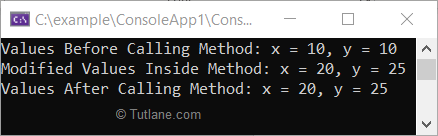## Differences between Ref and Out Keywords in C#

As discussed, the c# `ref` and `out` keywords are useful to pass method arguments as reference types and return multiple values from a function.

The following table lists the differences between ref and out in c#.

RefOut
The variables that are passing as arguments using the ref keyword must be initialized. It's not mandatory to initialize the variables to pass as arguments using out keyword.
It's not mandatory to initialize the variable before returning the value to the calling method. It's mandatory to initialize the variable in the method before returning the value to the calling method.
Useful to return multiple values from methods. Useful to return multiple values from methods.